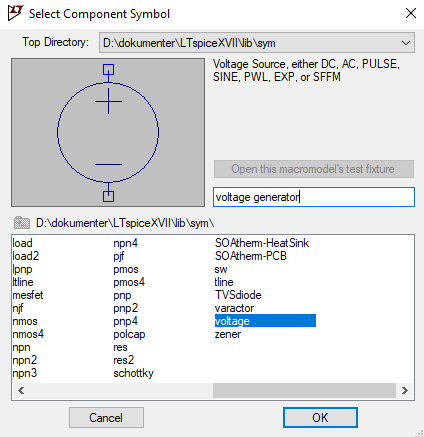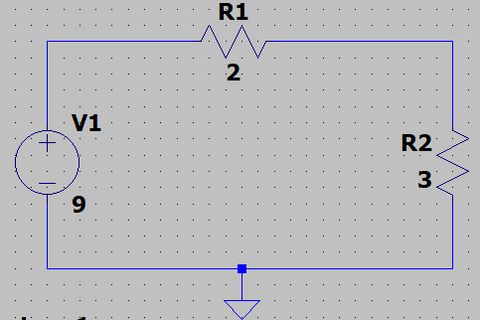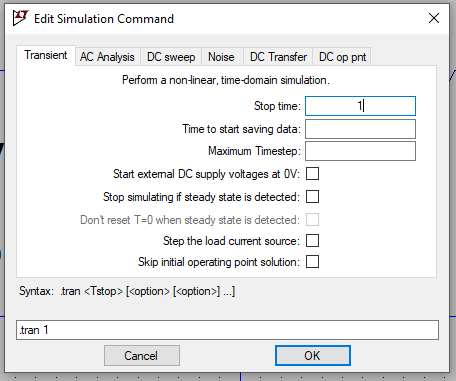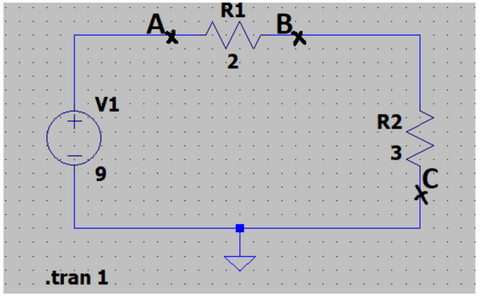# Introduction

So you want to develop your first circuit? Time to learn how to simulate! The ability to simulate circuits is an important tool for electronics makers, as it enables them to test and analyze their designs before building prototypes. This is important both for both time and money when developing a circuit.
In a simulation you can create your circuit and test it to see if it in theory performs as you meant it to. A widely used program for this is LT-Spice. Let’s take a look and see what it's all about.

## LT-Spice

Remember when we learned about basic circuit analysis in this blog?Figure 1: A circuit with the voltage generator V1 and two resistors R1 and R2.
V1 = 9 V
R1 = 2 Ω
R2 = 3 Ω

When we introduced this circuit, we calculated the voltage drops around the closed loop. Now we are going to simulate it and hopefully we will get the same results!

But wait. This LT-Spice.. How does one use it? Worry no longer for I will explain every step of the process and you’ll be simulating circuits in no time!

First step is to draw the circuit in the program, with all the right components and generators.

For that we open the program and start a new project.

- Press File in the top left corner

- Choose New Schematic (press Ctrl+N)

Now we should have the blank page, ready for us to draw our circuit in.

The two resistors can be drawn in the program by pressing r. You can rotate components by pressing Ctrl+R. Right click on the resistor symbols to adjust their values.

For implementing our voltage generator in our circuit press F2. The window 'Select Component Symbol' pops up. Search for Voltage and pick the voltage generator, see Figure 2. Right click on the voltage generator symbol to adjust its voltage.Figure 2: LT-Spice Select Component Symbol window.

The last we need now is Ground. For that we press g.

You can always click the scissor symbol at the menu at the top, to remove anything you might have drawn in your program that you don't want to be there.

Now we have all we need for our circuit and we just need to connect them to each other. Press F3 to connect your components and generators. Then drag your mouse from the points you want your connections, by pressing and holding left-click on your mouse.

When all our connections are made and we have insersted our values for our two resistors and the value for our voltage generator, we should have something that looks like this, see Figure 3.Figure 3

For simulating the drawn circuit, press the symbol with the running man, run, in the menu at the top.
The window 'Edit Simulation Command' pops up, see Figure 4. Don’t worry too much about all of these settings for now. We only need to input a Stop time. The amount of time doesn’t matter too much now either,  so we can press 1 for example. We have now told the program to simulate our circuit over the span of 1 second, see Figure 4.Figure 4: LT-Spice Edit Simulation Command window.

Then press ok and a graph should appear either below, besides or above your circuit.
This is the graph that shows the values for our simulations.

To measure the voltage across the resistor R1, drag your mouse from A to B - see Figure 5.Figure 5

The component you drag your mouse over is the component you measure the voltage across. So for R2 drag your mouse from B to C. A measurement should appear on your graph indicating the voltage across the given component over the span of a second. The name of this simulation has a color and a name on the graph. You can change the color for all simulations shown on the graph by right-clicking on their names. The names for the voltages on your graph starts with a V and the names for the current starts with an I.

To measure the current through a component click on the component you want to measure. Another measurement for the current should appear on the graph. (Notice that the left side measures the voltages on the vertical axis and the right side measures the currents or vice versa.)

We should now have something that looks like this, see Figure 6.Figure 6

The calculations we made in this blog, from which our circuit stems from, matches with our simulations. This means we have drawn the circuit into LT-Spice and measured the voltages and currents correctly. We now have an understanding of the basics of simulating circuits in LT-Spice!

In other words we can now simulate circuits to test how they operate in theory. And this is the goal of circuit simulation. It's easy to imagine how useful this is, especially when working with much bigger circuits with a lot of components. With LT-Spice we can quickly test the circuit, but it is important not to be too quick, as if somehow an error in the drawn circuit occurs, the simulations might be misguiding. So be sure to double check your circuits when simulating!
Fortunately for us LT-Spice is an quite intuitive program that you rapidly will get the hang of so you can construct many of your very own circuits. Circuit simulations can also be used to get an intuitive understanding of how different components affect the circuits, by adding, removing or changing values for the components. Circuit simulation is a great tool and a must for every electronic producer!

Skriv kommentar!